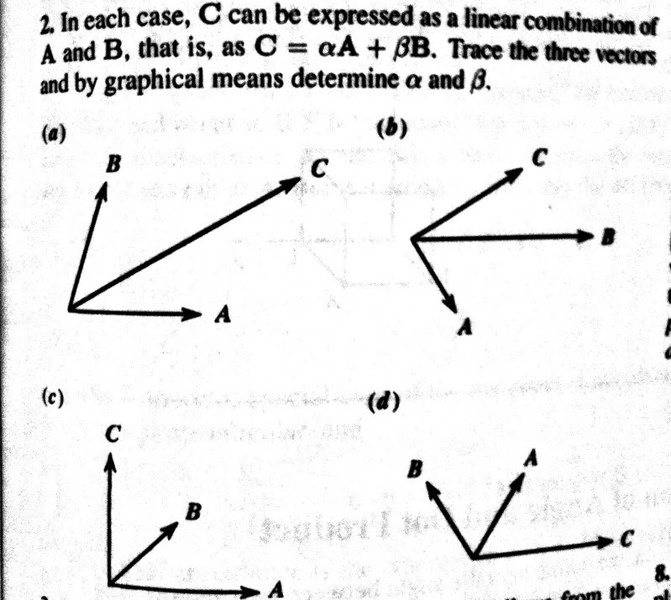# Graphical Vector Problem (What are they asking for?)

"Graphical" Vector Problem (What are they asking for?)

## Homework Statement

Here is the problem statement. I honestly don't understand what it is they are asking for? Do they want actual numbers? Am I supposed to measure the lengths with a ruler or something? I know it's easy once I interpret the question correctlyHow would you interpret this one?

LCKurtz
Homework Helper
Gold Member

Hey, that vector A in part (c) is bent. Must have a non-zero curl :tongue2:

Yup, that's what I would do. Draw them carefully, construct the corresponding parallelograms and figure out the alpha and beta by measuring to scale things. "Trace" them certainly suggests that.

Hey, that vector A in part (c) is bent. Must have a non-zero curl :tongue2:

Yup, that's what I would do. Draw them carefully, construct the corresponding parallelograms and figure out the alpha and beta by measuring to scale things. "Trace" them certainly suggests that.

Haha! :rofl: Thanks LCKurtz! Don't quit your day job (assuming you have one!)!

Mark44
Mentor

By "graphical means" they mean for you to extend the vectors A and B so that the lengthened vectors form two sides of a parallelogram whose diagonal is C. You'll need to find the lengths of A and B with a ruler so that you can determine the values of $\alpha$ and $\beta$ so that $\alpha$A + $\beta$B = C.

For example, if you have to double the lengths of A and B to form the parallelogram, then $\alpha$ = $\beta$ = 2.

I must be an idiot. I cannot see a way to extend or shorten A and B such that C becomes the diagonal of the parallelogram. My brain keeps making B the diagonal since it is more intuitive to me.

LCKurtz